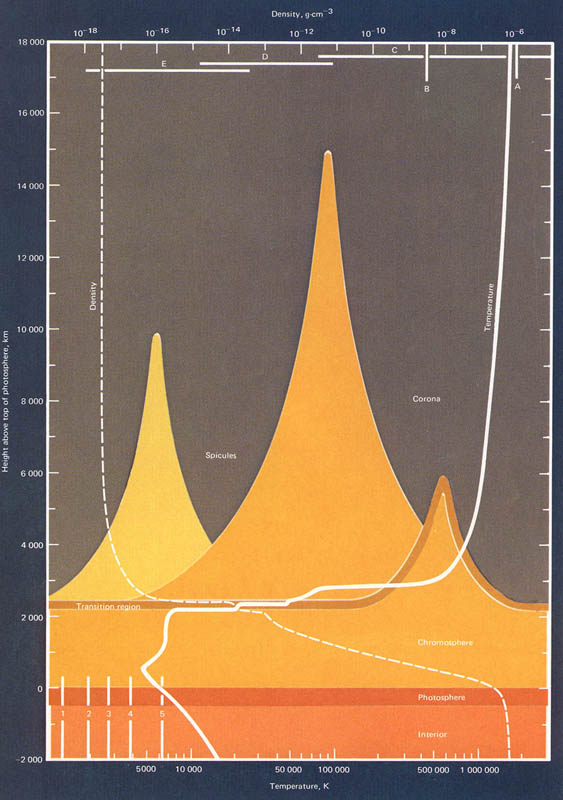What is the exact density of the Sun? It is made of hydrogen and helium, two of the lightest elements known. Does it mean it’s not very dense? What factors affect stellar density and how do we compute the Sun’s density? Does gravity have any effect at all? These are just some of the questions regarding the Sun and its density. One doesn’t have to be a scientist in order to understand and analyze it.

What is density in the first place? It is defined as the matter’s mass per unit volume. In layman terms it is how concentrated or jam-packed together a particular object is. It was Archimedes who discovered the principle behind density. It is computed by dividing mass over volume. How can we observe density in everyday life? For example there is a pond of water. Then imagine cotton and silver that has the same weight. Though they have the same weight, their density is different. This is why cotton, which has low density, would float while silver, which is denser, will not.

Now how about the density of the Sun? First, one should find out the mass of the Sun. The Sun’s mass is 2×1033 grams. The next thing to find out is the Sun’s volume. It is 1.41 x 1033 cm3. If one will apply the formula of 2×1033 grams divided by 1.41 x 1033 cm3, then the result would be 1.4 g/cm3. The density is high even if it is made of light gases, but of the gravity inside the Sun is strong, thus increasing the pressure.

So the Sun is a very dense star. The outer layer may seem less dense but upon further observation, the inner layer has high pressure due to gravity. Higher pressure increases matter density. It is because of these factors that the density of the Sun is 1.4 g/cm3.